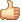# LDA-math-LDA 文本建模

5. LDA 文本建模

5.1 游戏规则LDA模型\begin{align*}
\overrightarrow{\mathbf{w}} & = (\overrightarrow{w}_1, \cdots, \overrightarrow{w}_M) \\
\overrightarrow{\mathbf{z}} & = (\overrightarrow{z}_1, \cdots, \overrightarrow{z}_M)
\end{align*}语料生成过程中的 word 和 topic

5.2 物理过程分解LDA概率图模型表示

•  $\overrightarrow{\alpha}\rightarrow \overrightarrow{\theta}_m \rightarrow z_{m,n}$, 这个过程表示在生成第$m$ 篇文档的时候，先从第一个坛子中抽了一个doc-topic 骰子 $\overrightarrow{\theta}_m$, 然后投掷这个骰子生成了文档中第 $n$ 个词的topic编号$z_{m,n}$；
•  $\overrightarrow{\beta} \rightarrow \overrightarrow{\varphi}_k \rightarrow w_{m,n} | k=z_{m,n}$, 这个过程表示用如下动作生成语料中第$m$篇文档的第 $n$个词：在上帝手头的$K$ 个topic-word 骰子 $\overrightarrow{\varphi}_k$ 中，挑选编号为 $k=z_{m,n}$的那个骰子进行投掷，然后生成 word $w_{m,n}$；$$p(\overrightarrow{z}_m |\overrightarrow{\alpha}) = \frac{\Delta(\overrightarrow{n}_m+\overrightarrow{\alpha})}{\Delta(\overrightarrow{\alpha})}$$

$$Dir(\overrightarrow{\theta}_m| \overrightarrow{n}_m + \overrightarrow{\alpha}).$$

\begin{align}
\label{corpus-topic-prob}
p(\overrightarrow{\mathbf{z}} |\overrightarrow{\alpha}) & = \prod_{m=1}^M p(\overrightarrow{z}_m |\overrightarrow{\alpha}) \notag \\
\end{align}\begin{align*}
\overrightarrow{\mathbf{w}}’ &= (\overrightarrow{w}_{(1)}, \cdots, \overrightarrow{w}_{(K)}) \\
\overrightarrow{\mathbf{z}}’ &= (\overrightarrow{z}_{(1)}, \cdots, \overrightarrow{z}_{(K)})
\end{align*}$$p(\overrightarrow{w}_{(k)} |\overrightarrow{\beta}) = \frac{\Delta(\overrightarrow{n}_k+\overrightarrow{\beta})}{\Delta(\overrightarrow{\beta})}$$

$$Dir( \overrightarrow{\varphi}_k| \overrightarrow{n}_k + \overrightarrow{\beta}).$$

\begin{align}
\label{corpus-word-prob}
p(\overrightarrow{\mathbf{w}} |\overrightarrow{\mathbf{z}},\overrightarrow{\beta}) &= p(\overrightarrow{\mathbf{w}}’ |\overrightarrow{\mathbf{z}}’,\overrightarrow{\beta}) \notag \\
&= \prod_{k=1}^K p(\overrightarrow{w}_{(k)} | \overrightarrow{z}_{(k)}, \overrightarrow{\beta}) \notag \\
\end{align}

\begin{align}
\label{lda-corpus-likelihood}
p(\overrightarrow{\mathbf{w}},\overrightarrow{\mathbf{z}} |\overrightarrow{\alpha}, \overrightarrow{\beta}) &=
p(\overrightarrow{\mathbf{w}} |\overrightarrow{\mathbf{z}}, \overrightarrow{\beta}) p(\overrightarrow{\mathbf{z}} |\overrightarrow{\alpha}) \notag \\
&= \prod_{k=1}^K \frac{\Delta(\overrightarrow{n}_k+\overrightarrow{\beta})}{\Delta(\overrightarrow{\beta})}
\end{align}

5.3 Gibbs Sampling

\begin{align*}
p(z_i = k|\overrightarrow{\mathbf{z}}_{\neg i}, \overrightarrow{\mathbf{w}}) \propto
p(z_i = k, w_i = t |\overrightarrow{\mathbf{z}}_{\neg i}, \overrightarrow{\mathbf{w}}_{\neg i}) \\
\end{align*}

1.  $\overrightarrow{\alpha} \rightarrow \overrightarrow{\theta}_m \rightarrow \overrightarrow{z}_{m}$
2. $\overrightarrow{\beta} \rightarrow \overrightarrow{\varphi}_k \rightarrow \overrightarrow{w}_{(k)}$

\begin{align*}
p(\overrightarrow{\theta}_m|\overrightarrow{\mathbf{z}}_{\neg i}, \overrightarrow{\mathbf{w}}_{\neg i})
&= Dir(\overrightarrow{\theta}_m| \overrightarrow{n}_{m,\neg i} + \overrightarrow{\alpha}) \\
p(\overrightarrow{\varphi}_k|\overrightarrow{\mathbf{z}}_{\neg i}, \overrightarrow{\mathbf{w}}_{\neg i})
&= Dir( \overrightarrow{\varphi}_k| \overrightarrow{n}_{k，\neg i} + \overrightarrow{\beta})
\end{align*}

=
=
=
=
=
=
=

\begin{align*}
p(z_i = k|\overrightarrow{\mathbf{z}}_{\neg i}, \overrightarrow{\mathbf{w}}) & \propto
p(z_i = k, w_i = t |\overrightarrow{\mathbf{z}}_{\neg i}, \overrightarrow{\mathbf{w}}_{\neg i}) \\
&= \int p(z_i = k, w_i = t, \overrightarrow{\theta}_m,\overrightarrow{\varphi}_k |
\overrightarrow{\mathbf{z}}_{\neg i}, \overrightarrow{\mathbf{w}}_{\neg i}) d \overrightarrow{\theta}_m d \overrightarrow{\varphi}_k \\
&= \int p(z_i = k, \overrightarrow{\theta}_m|\overrightarrow{\mathbf{z}}_{\neg i}, \overrightarrow{\mathbf{w}}_{\neg i})
\cdot p(w_i = t, \overrightarrow{\varphi}_k | \overrightarrow{\mathbf{z}}_{\neg i}, \overrightarrow{\mathbf{w}}_{\neg i})
d \overrightarrow{\theta}_m d \overrightarrow{\varphi}_k \\
&= \int p(z_i = k |\overrightarrow{\theta}_m) p(\overrightarrow{\theta}_m|\overrightarrow{\mathbf{z}}_{\neg i}, \overrightarrow{\mathbf{w}}_{\neg i})
\cdot p(w_i = t |\overrightarrow{\varphi}_k) p(\overrightarrow{\varphi}_k|\overrightarrow{\mathbf{z}}_{\neg i}, \overrightarrow{\mathbf{w}}_{\neg i})
d \overrightarrow{\theta}_m d \overrightarrow{\varphi}_k \\
&= \int p(z_i = k |\overrightarrow{\theta}_m) Dir(\overrightarrow{\theta}_m| \overrightarrow{n}_{m,\neg i} + \overrightarrow{\alpha}) d \overrightarrow{\theta}_m \\
& \hspace{0.2cm} \cdot \int p(w_i = t |\overrightarrow{\varphi}_k) Dir( \overrightarrow{\varphi}_k| \overrightarrow{n}_{k,\neg i} + \overrightarrow{\beta}) d \overrightarrow{\varphi}_k \\
&= \int \theta_{mk} Dir(\overrightarrow{\theta}_m| \overrightarrow{n}_{m,\neg i} + \overrightarrow{\alpha}) d \overrightarrow{\theta}_m
\cdot \int \varphi_{kt} Dir( \overrightarrow{\varphi}_k| \overrightarrow{n}_{k,\neg i} + \overrightarrow{\beta}) d \overrightarrow{\varphi}_k \\
&= E(\theta_{mk}) \cdot E(\varphi_{kt}) \\
&= \hat{\theta}_{mk} \cdot \hat{\varphi}_{kt} \\
\label{gibbs-sampling-deduction}
\end{align*}

\begin{align*}
\hat{\theta}_{mk} &= \frac{n_{m,\neg i}^{(k)} + \alpha_k}{\sum_{k=1}^K (n_{m,\neg i}^{(k)} + \alpha_k)} \\
\hat{\varphi}_{kt} &= \frac{n_{k,\neg i}^{(t)} + \beta_t}{\sum_{t=1}^V (n_{k,\neg i}^{(t)} + \beta_t)}
\end{align*}

\begin{equation}
\label{gibbs-sampling}
p(z_i = k|\overrightarrow{\mathbf{z}}_{\neg i}, \overrightarrow{\mathbf{w}}) \propto
\frac{n_{m,\neg i}^{(k)} + \alpha_k}{\sum_{k=1}^K (n_{m,\neg i}^{(k)} + \alpha_k)}
\cdot \frac{n_{k,\neg i}^{(t)} + \beta_t}{\sum_{t=1}^V (n_{k,\neg i}^{(t)} + \beta_t)}
\end{equation}doc-topic-word 路径概率

5.4 Training and Inference

• 估计模型中的参数 $\overrightarrow{\varphi}_1, \cdots, \overrightarrow{\varphi}_K$ 和 $\overrightarrow{\theta}_1, \cdots, \overrightarrow{\theta}_M$；
• 对于新来的一篇文档$doc_{new}$，我们能够计算这篇文档的 topic 分布$\overrightarrow{\theta}_{new}$。LDA 对于专业做机器学习的兄弟而言，只能算是一个简单的Topic Model。但是对于互联网中做数据挖掘、语义分析的工程师，LDA 的门槛并不低。 LDA 典型的属于这样一种机器学习模型：要想理解它，需要比较多的数学背景，要在工程上进行实现，却相对简单。 Gregor Heinrich 的LDA 模型科普文章 Parameter estimation for text analysis 写得非常的出色，这是学习 LDA 的必看文章。不过即便是这篇文章，对于工程师也是有门槛的。我写的这个科普最好对照 Gregor Heinrich 的这篇文章来看， 我用的数学符号也是尽可能和这篇文章保持一致。

What I cannot create, I do not understand.
— Richard Feynman

LDA数学八卦

LDA-math 的汇总， “LDA数学八卦.pdf” 我整理贴出来了， 希望对大家理解 LDA 有帮助。 文章标题挂上“八卦”两字， 因为八卦意味着形式自由、不拘束、可以天马行空，细节处理上也难免有不严谨的地方；当然我也希望八卦是相对容易理解的。

LDA数学八卦.pdf 下载

## 《LDA-math-LDA 文本建模》有22个想法

1.chu说道：

前排留名。等了好久终于等到了LDA。
为同学们节约搜索时间，plsa的连接如下
http://cs.brown.edu/~th/papers/Hofmann-UAI99.pdf
Blei的LDA也有简单的review PLSA，unigram model
http://www.cs.princeton.edu/~blei/papers/BleiNgJordan2003.pdf
08年Blei同学来了次回锅肉，把lda改进改进。在LDA里，topic之间是独立的，但是有时候应该是有影响的；所以，换另一个先验分布
http://www.cs.cmu.edu/~lafferty/pub/ctm.pdf

1.alphago说道：

其实还有一本书对LDA写的也很好更系统，还讲了其他资料没有的“变分推断”，这本书叫《LDA漫游指南》，希望能对你有用

2.alphago说道：

其实还有一本书对LDA写的更好更系统，还讲了其他资料没有的“变分推断”，这本书叫《LDA漫游指南》，http://yuedu.baidu.com/ebook/d0b441a8ccbff121dd36839a
这位作者写的非常艰辛，放在百度阅读上供大家分享给大家阅读。你也可以去看看，希望对你有帮助

2.liutiejun说道：

这几篇和LDA相关的文章写得确实是太好了，简单易懂！！！

3.林风琦说道：

写的太好了，收益匪浅

4.unluckwin说道：

这5篇文章的系列实在太好了 支持作者 我仔细拜读

5.pudding说道：

有朝一日希望老师介绍一下topicmodels包里的VEM方法和CTM方法~

6.taylor说道：

请问 为什么 α→θm 对应于 Dirichlet 分布？在5.2中的alpha和beta的向量分别代表什么意义呢？

7.vector说道：

太棒了

8.yu说道：

很棒，赞！

9.傅颖说道：

博主，请问您还记不记得哪篇文章中有提到BETA=0.01; ALPHA=50/T; 以及迭代次数的一个经验值范围。我现在在写论文需要一个理论支撑，但是一直找不到那文章。不知道您可不可以帮忙？

10.吴智勇说道：

模型的学习过程中 topic的数目是人工指定的?

11.汪华东说道：

写的挺好

12.圣洁水说道：13.alphago说道：

其实还有一本书对LDA写的更好更系统，还讲了其他资料没有的“变分推断”，这本书叫《LDA漫游指南》，http://yuedu.baidu.com/ebook/d0b441a8ccbff121dd36839a
这位作者写的非常艰辛，放在百度阅读上供大家分享给大家阅读。希望楼主文章中提及，不要抹杀其他作者的辛勤工作

14.郭梓鑫说道：

LDA训练得到的模型参数，在inference的时候是怎么使用的？从Algorithm 7来看，好像inference过程没用到前面训练出来的topic-word频率矩阵。

15.郭梓鑫说道：

LDA训练得到的模型参数在inference的时候是怎么使用的？从Algorithm 7来看，inference过程貌似没有用到前面训练得到的topic-word共现频率矩阵。

16.说道：

写！得！太！好！了！最好的中文lda材料没有之一！就是数学基础不太好，有些推导没看懂….